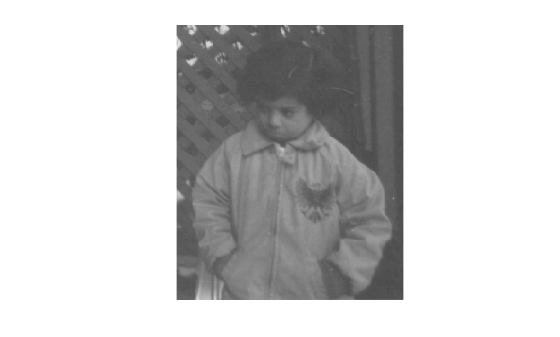# axes2pix

Convert axes coordinates to pixel coordinates

## Syntax

``pixelCoord = axes2pix(n,extent,axesCoord)``

## Description

example

````pixelCoord = axes2pix(n,extent,axesCoord)` converts an axes coordinate into an intrinsic ("pixel") coordinate. NoteThe `imref2d` object has several methods that facilitate conversion between intrinsic coordinates, world coordinates and array indices. ```

## Examples

collapse all

Display image.

`h = imshow('pout.tif');`Get the size of the image.

`[nrows,ncols] = size(get(h,'CData'));`

Get the image `XData` and `YData`.

`xdata = get(h,'XData')`
```xdata = 1×2 1 240 ```
`ydata = get(h,'YData')`
```ydata = 1×2 1 291 ```

Convert an axes coordinate into an intrinsic coordinate for the x and y dimensions.

`px = axes2pix(ncols,xdata,30)`
```px = 30 ```
`py = axes2pix(nrows,ydata,30)`
```py = 30 ```

Read an image and display it. Get the size of the image.

```I = imread('pout.tif'); [nrows,ncols] = size(I)```
```nrows = 291 ```
```ncols = 240 ```

Create a spatial referencing object for this image, with default property settings. By default, the upper-left corner of the image has intrinsic coordinate (1,1).

```RI = imref2d(size(I)); h = imshow(I,RI);````xData = get(h,'XData')`
```xData = 1×2 1 240 ```
`yData = get(h,'YData')`
```yData = 1×2 1 291 ```

For illustrative purposes, specify an arbitrary image extent in the x- and y-directions. This example shifts the image up by 20 pixels and to the right by 400 pixels. The example also shifts the image to the right by 100 pixels and compresses the image horizontally by a factor of 2.

```xWorldLimits = 0.5*xData + 400; yWorldLimits = yData - 20; RA = imref2d(size(I),xWorldLimits,yWorldLimits); imshow(I,RA)```Select a pixel, such as a pixel near the nose of the child. This pixel occurs around the axes coordinate (x, y) = (450, 90) in the modified image.

Convert the axes coordinate to an intrinsic coordinate.

`px = axes2pix(ncols,xWorldLimits,450)`
```px = 100 ```
`py = axes2pix(nrows,yWorldLimits,90)`
```py = 110 ```

The intrinsic coordinate of the point is at (100, 110). This agrees with the location of the nose in the original image.

## Input Arguments

collapse all

Number of image rows or columns, specified as a positive integer. `n` is the number of image columns for the x-coordinate, or the number of image rows for the y-coordinate.

Image world extent, specified as a 2-element numeric vector. `extent` is returned by `get(image_handle,'XData')` or `get(image_handle,'YData')`.

Axes coordinate to convert to intrinsic coordinates, specified as a numeric vector.

## Output Arguments

collapse all

Intrinsic coordinates, returned as a numeric vector.

Data Types: `double`

## Tips

• `axes2pix` performs minimal checking on the validity of the `n`, `axesCoord`, or `extent` arguments. For example, `axes2pix` can extrapolate from `extent` to return a negative coordinate. The function calling `axes2pix` bears responsibility for error checking.

## Version History

Introduced before R2006a